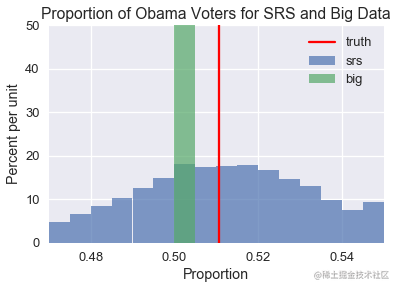# 数据科学的原理与技巧 二、数据生成

## Dewey 击败了 Truman

### 1948 年：盖洛普（Gallup）民意调查## 概率抽样

### 简单随机抽样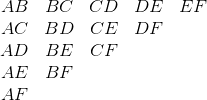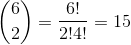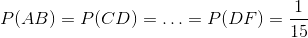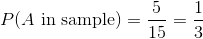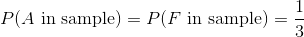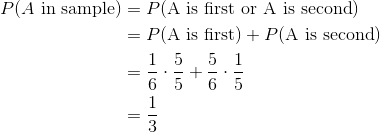### 整群抽样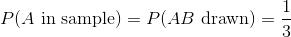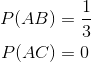### 分层抽样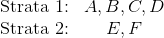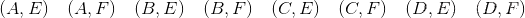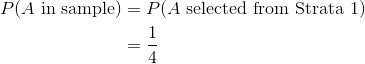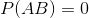## SRS vs “大数据”

# HIDDEN
total = 129085410
obama_true_count = 65915795
romney_true_count = 60933504
obama_true = obama_true_count / total
romney_true = romney_true_count / total

# 1 percent off
obama_big = obama_true - 0.01
romney_big = romney_true + 0.01复制代码

pd.DataFrame({
'truth': [obama_true, romney_true],
'big': [obama_big, romney_big],
}, index=['Obama', 'Romney'], columns=['truth', 'big']).plot.bar()
plt.title('Truth compared to a big non-random dataset')
plt.xlabel('Candidate')
plt.ylabel('Proportion of popular vote')
plt.ylim(0, 0.75)
None复制代码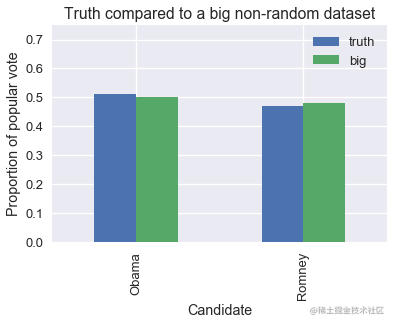srs_size = 400
big_size = 60000000
replications = 10000

def resample(size, prop, replications):
return np.random.binomial(n=size, p=prop, size=replications) / size

srs_simulations = resample(srs_size, obama_true, replications)
big_simulations = resample(big_size, obama_big, replications)复制代码

bins = bins=np.arange(0.47, 0.55, 0.005)
plt.hist(srs_simulations, bins=bins, alpha=0.7, normed=True, label='srs')
plt.hist(big_simulations, bins=bins, alpha=0.7, normed=True, label='big')

plt.title('Proportion of Obama Voters for SRS and Big Data')
plt.xlabel('Proportion')
plt.ylabel('Percent per unit')
plt.xlim(0.47, 0.55)
plt.ylim(0, 50)
plt.axvline(x=obama_true, color='r', label='truth')
plt.legend()
None复制代码